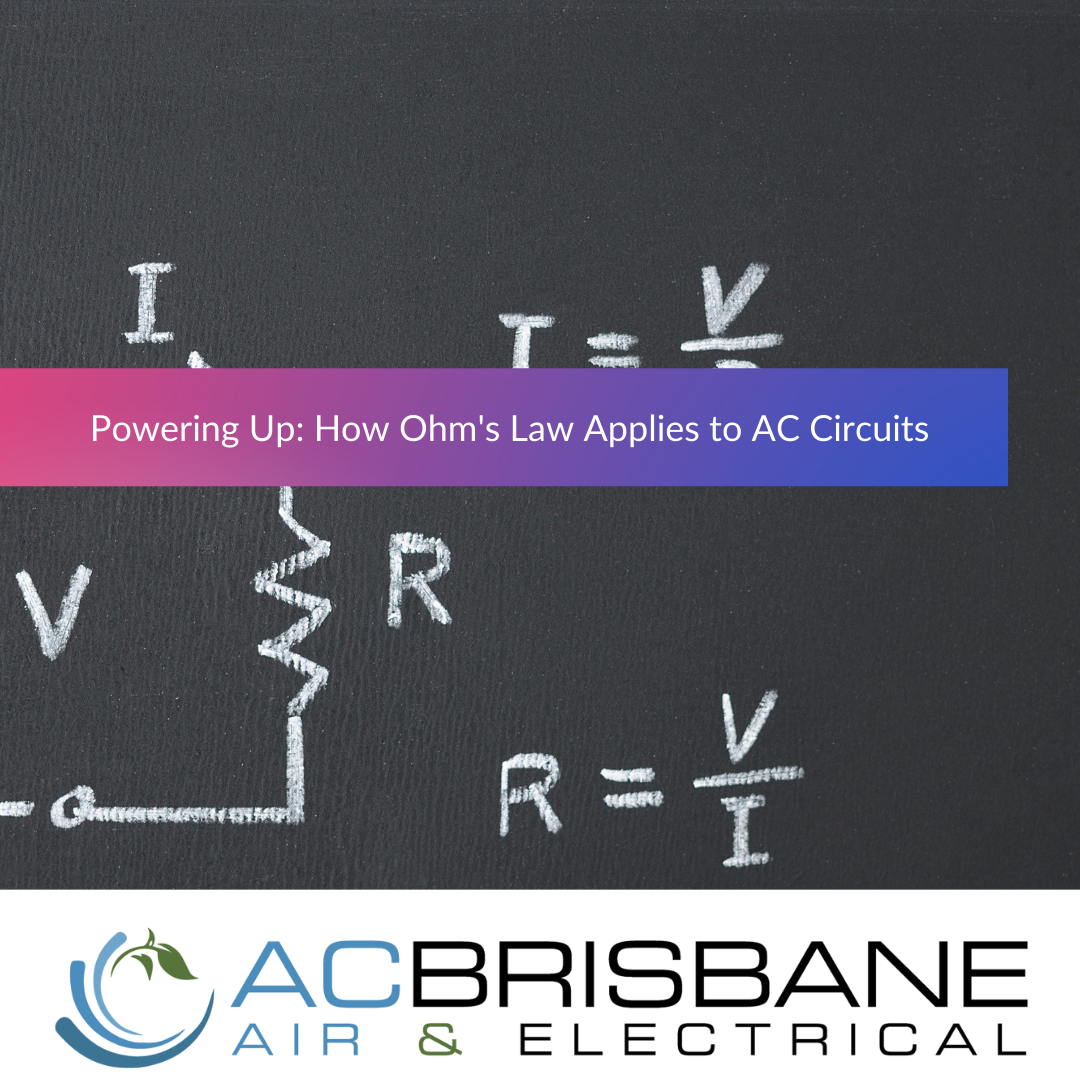# Powering Up: How Ohm's Law Applies to AC Circuits

Ohm's Law is widely known as a fundamental principle of electricity, which states that the current flowing through a conductor is directly proportional to the voltage across it and inversely proportional to the resistance of the conductor. However, Ohm's Law is only valid for direct current (DC) circuits. When it comes to alternating current (AC) circuits, the relationship between voltage, current and resistance is not as straightforward. In AC circuits, the resistance is not a constant value, but it changes with the frequency of the current.
For AC circuits, Ohm's Law is modified to include the concept of impedance. Impedance is the total opposition to current flow in an AC circuit, and it is represented by the letter "Z". Impedance is a complex quantity, which includes both resistance and reactance. Reactance is the opposition to current flow due to the inductive or capacitive nature of the circuit.
Ohm's Law for AC circuits can be represented by the following formula: V = Z.I
Where V is the voltage, Z is the impedance and I is the current. The formula shows that the voltage across an AC circuit is equal to the product of the impedance and the current.
A common example of Ohm's Law in AC circuits can be found in a toaster. A toaster typically operates on an AC voltage of 240 volts and has a power rating of around 800 watts. To calculate the current flowing through the toaster, we can use the formula I = P/V, where P is the power rating and V is the voltage. This gives us a current of around 3.3 amperes.
The impedance of the toaster can be calculated by using the formula Z = V/I, which gives us a value of around 72 ohms. This value can be used to determine the power factor of the toaster. Power factor is the ratio of actual power to apparent power and it is a measure of how efficiently the toaster is using the power supplied to it.